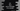# C# program to find the sum of all digits of a number## C# program to find the sum of all digits of a number:

In this post, we will learn how to find the sum of all digits of a number. The number will be provided by the user and it will find the sum of all digits.

### Algorithm to use:

To find the sum of all digits of a number, we need to find all the digits of the number. For that, we need to keep dividing the number by 10 and the remainder is the last number.

For example, for the number 123,

• 123 % 10 -> it is 3. change 123 to 123/10 -> 12
• 12 % 10 -> it is 2. change 12 to 12/10 -> 1
• 1 % 10 -> it is 1. change 1 to 1/10 -> 0. Stop.

We will use the below algorithm to find the sum of digits of that number:

• Get the number from the user
• Initialize a variable sum with
• Find the number % 10 i.e. the remainder if we divide the number by 10.
• Change the number to number / 10.
• Keep doing until the value of number becomes 0.

### C# program:

Below is the complete C# program:

``````using System;

public class Program {
static int findSum(int no) {
int remainder;
int sum = 0;

while (no > 0) {
remainder = no % 10;
sum = sum + remainder;
no = no / 10;
}

return sum;
}

public static void Main(string[] args) {
int number;

Console.WriteLine("Enter a number: ");

Console.Write("Sum of the digits " + findSum(number));
}
}``````

Here,

• It is asking the user to enter a number. The number is read and stored in the number variable.
• findSum method is used to find the sum of digits of the number. It takes one number as the parameter and returns the sum of its digits.
• remainder is used to store the remainder after dividing the number by 10.
• It uses a while loop that adds the digits of the number. sum is used to store the sum of all digits. It is initialized as 0. Inside the loop, it is adding the remainder value.
• This while loop will run until the value of no becomes 0.

### Output:

It will print output as like below:

``````Enter a number:
1245
Sum of the digits 12``````